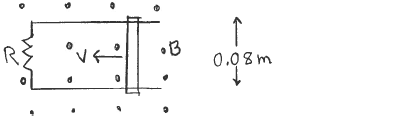# Problem: A conducting rod with length 0.0800 m slides without friction on conducting metal rails, as shown in the sketch. The apparatus is in a uniform magnetic field that has magnitude B = 0.600 T and that is directed out of the page. The resistance of the circuit is a constant R = 12.0 Ω. What is the magnitude of the external force required to move the bar at a constant speed of 5.00 m/s?

###### FREE Expert Solution
90% (496 ratings)
###### Problem Details

A conducting rod with length 0.0800 m slides without friction on conducting metal rails, as shown in the sketch. The apparatus is in a uniform magnetic field that has magnitude B = 0.600 T and that is directed out of the page. The resistance of the circuit is a constant R = 12.0 Ω. What is the magnitude of the external force required to move the bar at a constant speed of 5.00 m/s?Frequently Asked Questions

What scientific concept do you need to know in order to solve this problem?

Our tutors have indicated that to solve this problem you will need to apply the !! Review: Faraday's + Lenz's Laws concept. If you need more !! Review: Faraday's + Lenz's Laws practice, you can also practice !! Review: Faraday's + Lenz's Laws practice problems.

How long does this problem take to solve?

Our expert Physics tutor, Juan took 5 minutes and 15 seconds to solve this problem. You can follow their steps in the video explanation above.